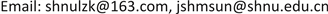﻿ 基于因子分析法的中国商业银行财务竞争力研究 Research on Financial Competitiveness of Chinese Commercial Banks Based on Factor Analysis

Finance
Vol. 09  No. 02 ( 2019 ), Article ID: 28774 , 8 pages
10.12677/FIN.2019.92009

Research on Financial Competitiveness of Chinese Commercial Banks Based on Factor Analysis

Zhongkun Lu, Hongmei Sun

Business College of Shanghai Normal University, ShanghaiReceived: Jan. 9th, 2019; accepted: Jan. 26th, 2019; published: Feb. 2nd, 2019ABSTRACT

Based on the semi-annual report and quarterly financial data publicly disclosed by 16 listed commercial banks in China in 2018, this paper selects nine bank financial indicators as research samples, and uses the factor analysis method in statistical analysis software SPSS 22.0 to finance the banks. Compare competitiveness, combine different factors to analyze the competitive advantages of each bank, and provide reasonable suggestions for banks to further enhance their competitiveness.

Keywords:Commercial Bank, Factor Analysis, CompetitivenessCopyright © 2019 by author(s) and Hans Publishers Inc.1. 引言

2. 文献综述

2.1. 核心竞争力指标分析

2.2. 经营绩效分析

2.3. 现阶段我国商业银行优劣势分析

3. 样本数据和关键财务指标选择

3.1. 指标选取Table 1. Core competitiveness index evaluation system

3.2. 数据来源

4. 财务竞争力实证结果及分析

4.1. KMO值检验

4.2. 总方差解释

4.3. 因子载荷矩阵

$\text{X1}=0.937{f}_{1}-0.053{f}_{2}+0.162{f}_{3}$

$\text{X2}=0.240{f}_{1}+0.631{f}_{2}+0.038{f}_{3}$

$\text{X3}=0.435{f}_{1}+0.809{f}_{2}+0.297{f}_{3}$

$\text{X4}=-0.312{f}_{1}-0.067{f}_{2}+0.680{f}_{3}$

$\text{X5}=0.424{f}_{1}+0.837{f}_{2}+0.090{f}_{3}$

$\text{X6}=0.442{f}_{1}-0.053{f}_{2}-0.700{f}_{3}$

$\text{X7}=-0.176{f}_{1}+0.655{f}_{2}-0.432{f}_{3}$

$\text{X8}=0.816{f}_{1}-0.531{f}_{2}+0.006{f}_{3}$

$\text{X9}=0.902{f}_{1}-0.286{f}_{2}+0.124{f}_{3}$

4.4. 因子的命名解释

4.5. 成分得分系数矩阵

$f1=0.305\text{X}1-0.025\text{X}2+0.054\text{X}3+0.061\text{X}4+0.005\text{X}5-0.010\text{X}6-0.242\text{X}7+0.316\text{X}8+0.325\text{X}9$

$f2=0.115\text{X}1+0.259\text{X}2+0.381\text{X}3+0.032\text{X}4+0.362\text{X}5-0.062\text{X}6+0.154\text{X}7-0.091\text{X}8+0.023\text{X}9$

$f3=-0.028\text{X}1+0.010\text{X}2-0.159\text{X}3-0.537\text{X}4-0.006\text{X}5+0.563\text{X}6+0.318\text{X}7+0.065\text{X}8-0.009\text{X}9$

$F=0.32143f1+0.28820f2+0.16195f3$

4.6. 各银行得分排名表Table 9. Summary table of factor scores and comprehensive scores of various commercial banks

Research on Financial Competitiveness of Chinese Commercial Banks Based on Factor Analysis[J]. 金融, 2019, 09(02): 83-90. https://doi.org/10.12677/FIN.2019.92009

1. 1. 陈一洪. 城市商业银行竞争力分析(2009-2016)——基于横向对比与动态发展的视角[J]. 新金融, 2017(9): 47-53.

2. 2. 中国农业银行江苏省分行、中国人民银行南京分行联合课题组, 张更生. 基于因子分析的我国上市银行财务竞争力研究[J]. 金融会计, 2017(3): 41-48.

3. 3. 刘小驹, 李小江. 商业银行竞争力实证分析及对策研究[J]. 农村金融研究, 2016(6): 41-45.

4. 4. 章颖薇, 胡小东. 基于层次分析法下两岸商业银行竞争力比较研究[J]. 亚太经济, 2014(4): 91-96.

5. 5. 张虎, 潘攀, 范莉莉. 效率视角下商业银行竞争力评价与预测研究[J]. 软科学, 2016, 30(2): 130-133.

6. 6. 上官飞, 舒长江. 基于因子分析的中国商业银行绩效评价[J]. 经济问题, 2011(1): 109-111.

7. 7. 张国富, 朱涛. 基于熵权因子分析法的商业银行运营绩效研究——以沪深25家为例[J]. 时代金融, 2017(30): 73-74.

8. 8. 刘晓蕾. 基于因子分析法分析我国上市商业银行的经营绩效[J]. 商场现代化, 2018(22): 121-124.

9. 9. 朱红杰. 上市商业银行财务竞争力综合评分分析[J]. 金融理论与实践, 2016(7): 107-111.

10. 10. 孙龙建, 李祥茂.我国商业银行综合绩效的因子分析——基于2012年年报的数据[J]. 经济问题探索, 2014(3): 131-134.

11. 11. 冯帆, 温万祥. 基于因子分析法的中国上市商业银行财务绩效评价实证研究——以16家上市银行2015年年报数据为基础[J]. 当代经济, 2017(15): 133-135.